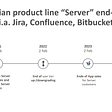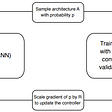# Diameter of Tree

For diameter of tree, we have a general workable solution which is from an arbitary node to reach the furthest node say f1. Then from f1 to reach another furthest node say f2. The distance between f1 and f2 will be the diameter.

Below is the implementation.

`# Definition for a binary tree node.# class TreeNode:#     def __init__(self, x):#         self.val = x#         self.left = None#         self.right = Noneclass Solution:    def diameterOfBinaryTree(self, root: TreeNode) -> int:        if root is None:return 0        d = {} # {node : parent, ...}        def bfs(parent=None):            Q = collections.deque([(root, None, 0)])            longest_node = None            while Q:                node, parent, level = Q.popleft()                longest_node = node                d[node] = parent                if node.left:                    Q.append((node.left, node, level+1))                if node.right:                    Q.append((node.right, node, level+1))            return longest_node        longest_node = bfs()        def bfs1(longest_node):            Q = collections.deque([(longest_node, 0)])            seen = {longest_node}            max_level = 0            while Q:                node, level = Q.popleft()                max_level = level                for new_node in [node.left, node.right, d[node]]:                    if new_node is not None and new_node not in seen:                        seen.add(new_node)                        Q.append((new_node, level+1))            return max_level        return bfs1(longest_node)`

However, the binary trees can be simpler.

`# Definition for a binary tree node.# class TreeNode:#     def __init__(self, x):#         self.val = x#         self.left = None#         self.right = Noneclass Solution:    def diameterOfBinaryTree(self, root: TreeNode) -> int:        if root is None:return 0        self.ans = 0        def helper(node):            if node is None:return 0            left = helper(node.left)            right = helper(node.right)            self.ans = max(self.ans, left+right+1)            return max(left, right) + 1        helper(root)        return self.ans-1`

1245. Tree Diameter

`class Solution:    def treeDiameter(self, edges: List[List[int]]) -> int:        c = collections.defaultdict(list)        for a,b in edges:            c[a].append(b)            c[b].append(a)        leaf = [key for key in c if len(c[key])==1]        def bfs(node):            open_list = [(node, 0)]            seen = set()            for node, d in open_list:                seen.add(node)                for new_node in c[node]:                    if new_node not in seen:                        open_list.append((new_node, d+1))            return open_list[-1]        end_node, _ = bfs(leaf)        return bfs(end_node)`

Or using deque

`class Solution:    def treeDiameter(self, edges: List[List[int]]) -> int:        graph = collections.defaultdict(list)        if not edges:return 0        for u, v in edges:            graph[u].append(v)            graph[v].append(u)        def bfs(node):            Q = collections.deque([(node, 0)])            node, level = None, 0            seen = {node}            while Q:                node, level = Q.popleft()                for next_node in graph[node]:                    if next_node not in seen:                        seen.add(next_node)                        Q.append((next_node, level+1))            return node, level        end, _ = bfs(edges)        _, level = bfs(end)        return level`

--

--

--

## More from Jimmy Shen

Data Scientist/MLE/SWE @takemobi

Love podcasts or audiobooks? Learn on the go with our new app.

## [ExpUp] My very first load testing## Why the Atlassians “Journey to cloud” spells problems for Customers## Minima Incentivized Node## Introduction to Elli Engineering: Our Guiding Principles## Ionic + Angular: Powering the App store and the web## Assignment 9 : Mobile App## Jimmy Shen

Data Scientist/MLE/SWE @takemobi

## Algorithm for Google Search Engine : PageRank Algorithm## Kth Smallest Element in a BST: Leetcode Medium — Blind 75 (Trees)## Neural Architecture Search (NAS)